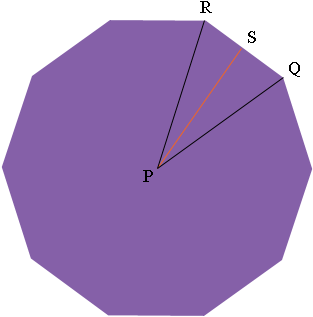SEARCH HOMEMath Central Quandaries & Querieshow do i find the apothem and area of a decagon that has a perimeter of 60?Hi Heather,

If you join each vertex of a regular decagon to the centre you subdivide the decagon into 10 congruent triangles. Thus if you find the area of one of the triangles and multiply by 10 you will have the area of the decagon. Let's look at one of these triangles, PQR in the diagram.S is the midpoint of QR ad hence angle QSR is a right angle. The measure of angle RPQ is 360/10 = 36 degrees and hence the measure of angle SPQ is 36/2 = 18 degrees. Since the perimeter is 60 units you can find the length of QS and hence the length of SP, the apothem, since

tan(SPQ) = |QS|/|SP|.

PennyMath Central is supported by the University of Regina and The Pacific Institute for the Mathematical Sciences.